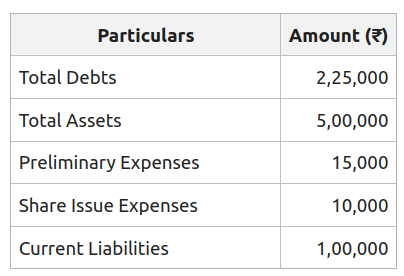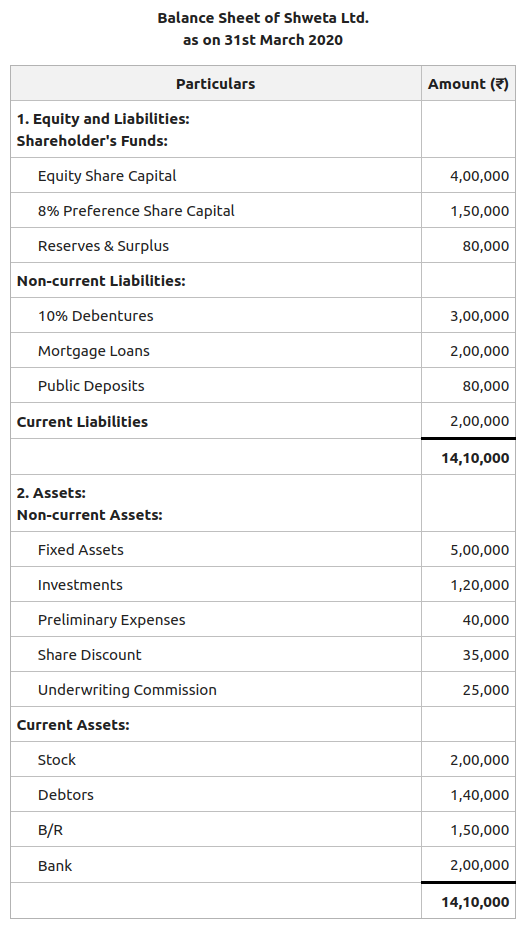Open in App
Not now

# Total Assets to Debt Ratio: Meaning, Formula and Examples

• Last Updated : 21 Oct, 2022

Financial Statements are prepared to know the profitability and financial position of the business in the market. These financial statements are then analysed with the help of different tools and methods. Ratio Analysis is one of the methods to analyse financial statements. The relationship between various financial factors of a business is defined through ratio analysis.

### What is Total Assets to Debt Ratio?

Total Assets to Debt Ratio is the ratio, through which the total assets of a company are expressed in relation to its long-term debts. It is a variation of the debt-equity ratio and gives the same indication as the debt-equity ratio.

Total Assets: Total Assets consists of all fixed and current assets of an organisation; however, it does not include the fictitious assets that appear on the asset side of the Balance Sheet. The fictitious assets include Share Issue Expenses, Preliminary Expenses, Underwriting Commission, Discount on Issue, etc., and the negative balance of Profit & Loss Statement.

Long-term Loans: Long-term Loans consist of long-term liabilities maturing after one year. The long-term liabilities of a company include all the long-term debts like Mortgage Loans, Debentures, Bank Loan, Public Deposits, etc.

The Total Assets to Debt Ratio is usually expressed as a pure ratio; i.e., 1:1 or 2:1.

### FormulaOrWhere,

Total Assets = Non-Current Assets (Tangible Assets + Intangible Assets + Non-Current Investments + Long Term Loans & Advances) +Current Assets

Debt = Long-Term Borrowings + Long-Term Provisions

### Significance

The Total Assets to Debt Ratio establishes a relationship between total assets and long-term loans. It also indicates the safety margin available to the firm’s long-term loans. In simple terms, it shows the extent to which the long-term loans of a company are covered by its total assets. A higher total assets to debt ratio represents more security to the lenders of long-term loans. However, lower total assets to debt ratio represent less security to the lenders of long-term loans, which indicates more dependence of the firm on long-term borrowed funds.

#### Illustration 1:

Compute Total Assets to Debt Ratio from the following information:#### Solution:OrTotal Assets = Non-Current Assets (Tangible Assets + Intangible Assets + Non-Current Investments + Long Term Loans & Advances) +Current Assets

Debt = Long Term Borrowings + Long Term Provisions

In the given question,

Net Total Assets = Total Assets – Fictitious Assets (Preliminary Expenses + Share Issue Expenses)

= ₹5,00,000 – (₹15,000 + ₹10,000)

= ₹5,00,000 – ₹25,000

= ₹4,75,000

Long-term Loans = Total Debts – Current Liabilities

= ₹2,25,000 – ₹1,00,000

= ₹1,25,000

Total Assets to Debt Ratio == 3.8:1

Comment:

Total Asset to Debt Ratio of 3.8:1 means that the company’s total assets are 3.8 times of its long-term loans. It indicates that the assets are sufficiently large and provides an adequate safety margin to the providers of long-term loan.

#### Illustration 2:

Compute Total Assets to Debt Ratio from the following Balance Sheet of Shweta Ltd. as on 31st March 2020:#### Solution:Total Assets = Non-Current Assets (Tangible Assets + Intangible Assets + Non-Current Investments + Long Term Loans & Advances) +Current Assets

Debt = Long-Term Borrowings + Long-Term Provisions

In the given question,

Total Assets = Fixed Assets + Investments + Stock + Debtors + B/R + Bank

= ₹5,00,000 + ₹1,20,000 + ₹2,00,000 + ₹1,40,000 + ₹1,50,000 + ₹2,00,000

= ₹13,10,000

Or,

Total Assets = Total Assets – (Preliminary Expenses + Share Discount  + Underwriting Commission)

= ₹14,10,000 – (₹40,000 + ₹35,000 + ₹25,000)

= ₹14,10,000 – ₹1,00,000

= ₹13,10,000

Debt = Mortgage Loans + 10% Debentures + Public Deposits

= ₹2,00,000 + ₹3,00,000 + ₹80,000

=₹5,80,000

Total Assets to Debt Ratio == 2.25:1

#### Comment:

Total Asset to Debt Ratio of 2.25:1 means that the company’s total assets are 2.25 times of its long-term loans. It indicates that the assets of Shweta Ltd. are sufficiently large and provides an adequate safety margin to the providers of long-term loan.

My Personal Notes arrow_drop_up
Related Articles